# Using Recursion 2004 Goodrich Tamassia Using Recursion 1

• Slides: 23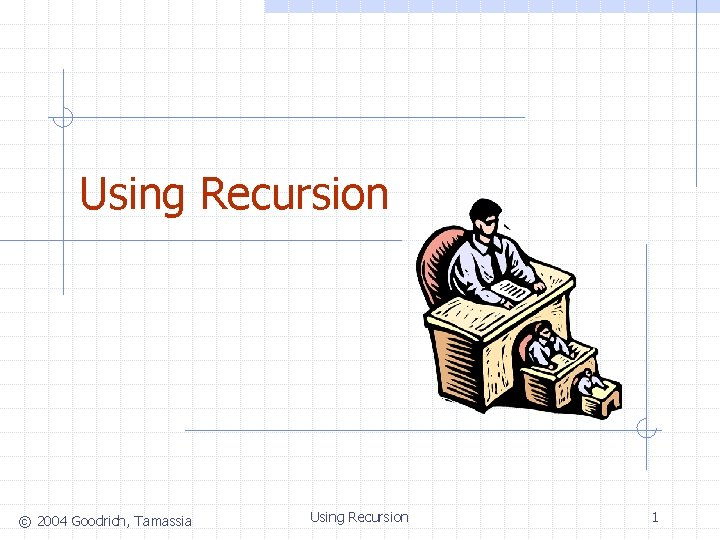Using Recursion © 2004 Goodrich, Tamassia Using Recursion 1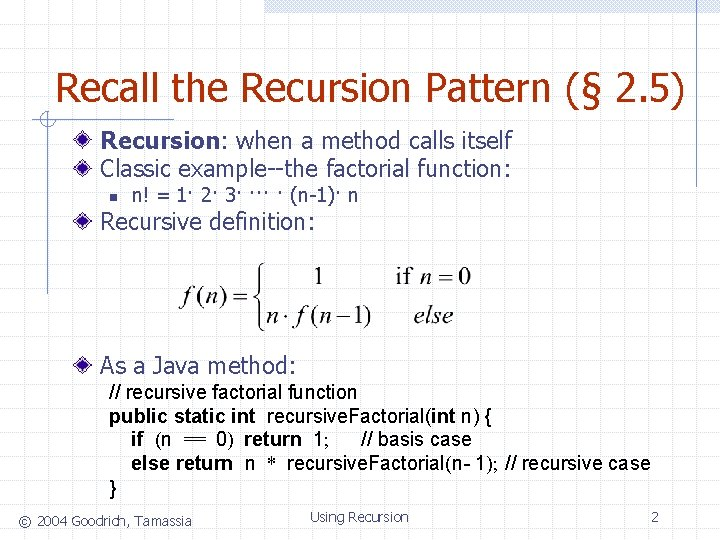Recall the Recursion Pattern (§ 2. 5) Recursion: when a method calls itself Classic example--the factorial function: n n! = 1· 2· 3· ··· · (n-1)· n Recursive definition: As a Java method: // recursive factorial function public static int recursive. Factorial(int n) { if (n == 0) return 1; // basis case else return n * recursive. Factorial(n- 1); // recursive case } © 2004 Goodrich, Tamassia Using Recursion 2Content of a Recursive Method Base case(s). n n Values of the input variables for which we perform no recursive calls are called base cases (there should be at least one base case). Every possible chain of recursive calls must eventually reach a base case. Recursive calls. n n Calls to the current method. Each recursive call should be defined so that it makes progress towards a base case. © 2004 Goodrich, Tamassia Using Recursion 3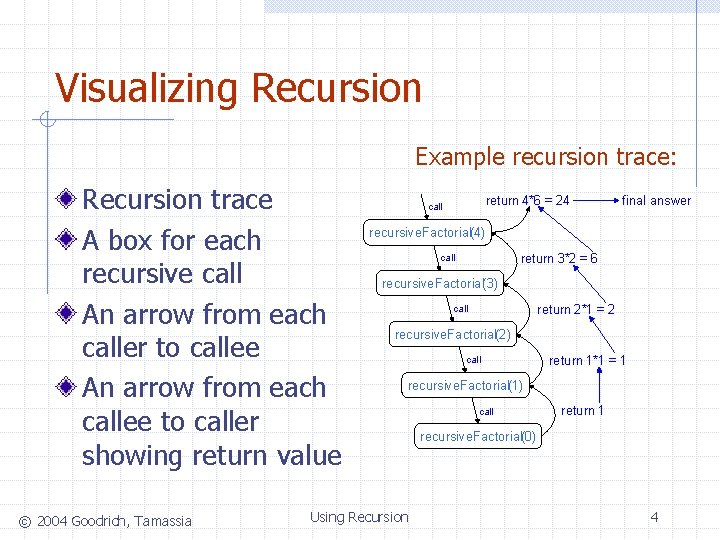Visualizing Recursion Example recursion trace: Recursion trace A box for each recursive call An arrow from each caller to callee An arrow from each callee to caller showing return value © 2004 Goodrich, Tamassia return 4*6 = 24 call final answer recursive. Factorial(4) return 3*2 = 6 call recursive. Factorial(3) return 2*1 = 2 call recursive. Factorial(2) call return 1*1 = 1 recursive. Factorial(1) Using Recursion call return 1 recursive. Factorial(0) 4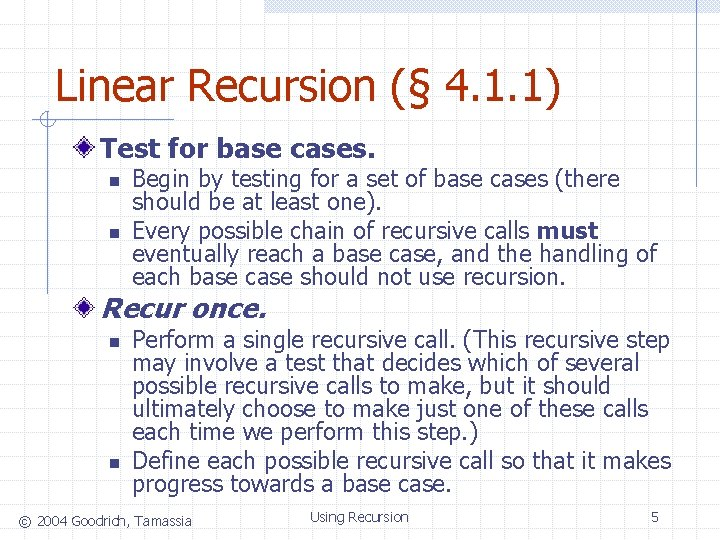Linear Recursion (§ 4. 1. 1) Test for base cases. n n Begin by testing for a set of base cases (there should be at least one). Every possible chain of recursive calls must eventually reach a base case, and the handling of each base case should not use recursion. Recur once. n n Perform a single recursive call. (This recursive step may involve a test that decides which of several possible recursive calls to make, but it should ultimately choose to make just one of these calls each time we perform this step. ) Define each possible recursive call so that it makes progress towards a base case. © 2004 Goodrich, Tamassia Using Recursion 5A Simple Example of Linear Recursion Algorithm Linear. Sum(A, n): Example recursion trace: Input: A integer array A and an integer n = 1, such that A has at least n elements Output: call Linear. Sum (A, 5) call The sum of the first n integers in A if n = 1 then return A else return Linear. Sum(A, n - 1) + A[n - 1] © 2004 Goodrich, Tamassia return 15 + A = 15 + 5 = 20 Using Recursion return 13 + A = 13 + 2 = 15 Linear. Sum (A, 4) call return 7 + A = 7 + 6 = 13 Linear. Sum (A, 3) call return 4 + A = 4 + 3 = 7 Linear. Sum (A, 2) call return A = 4 Linear. Sum (A, 1) 6Reversing an Array Algorithm Reverse. Array(A, i, j): Input: An array A and nonnegative integer indices i and j Output: The reversal of the elements in A starting at index i and ending at j if i < j then Swap A[i] and A[ j] Reverse. Array(A, i + 1, j - 1) return © 2004 Goodrich, Tamassia Using Recursion 7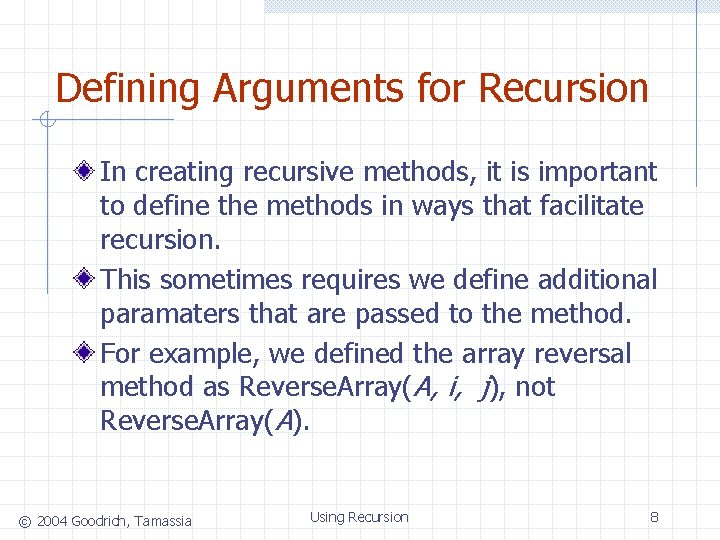Defining Arguments for Recursion In creating recursive methods, it is important to define the methods in ways that facilitate recursion. This sometimes requires we define additional paramaters that are passed to the method. For example, we defined the array reversal method as Reverse. Array(A, i, j), not Reverse. Array(A). © 2004 Goodrich, Tamassia Using Recursion 8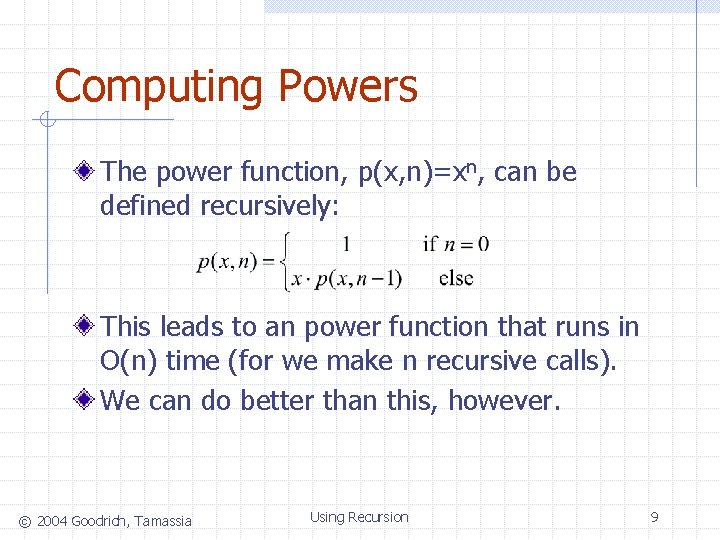Computing Powers The power function, p(x, n)=xn, can be defined recursively: This leads to an power function that runs in O(n) time (for we make n recursive calls). We can do better than this, however. © 2004 Goodrich, Tamassia Using Recursion 9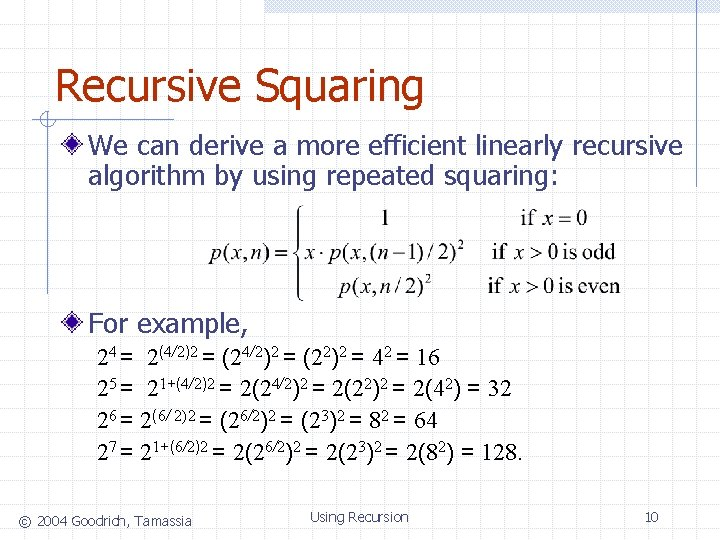Recursive Squaring We can derive a more efficient linearly recursive algorithm by using repeated squaring: For example, 24 = 2(4/2)2 = (22)2 = 42 = 16 25 = 21+(4/2)2 = 2(22)2 = 2(42) = 32 26 = 2(6/ 2)2 = (26/2)2 = (23)2 = 82 = 64 27 = 21+(6/2)2 = 2(23)2 = 2(82) = 128. © 2004 Goodrich, Tamassia Using Recursion 10A Recursive Squaring Method Algorithm Power(x, n): Input: A number x and integer n = 0 Output: The value xn if n = 0 then return 1 if n is odd then y = Power(x, (n - 1)/ 2) return x · y ·y else y = Power(x, n/ 2) return y · y © 2004 Goodrich, Tamassia Using Recursion 11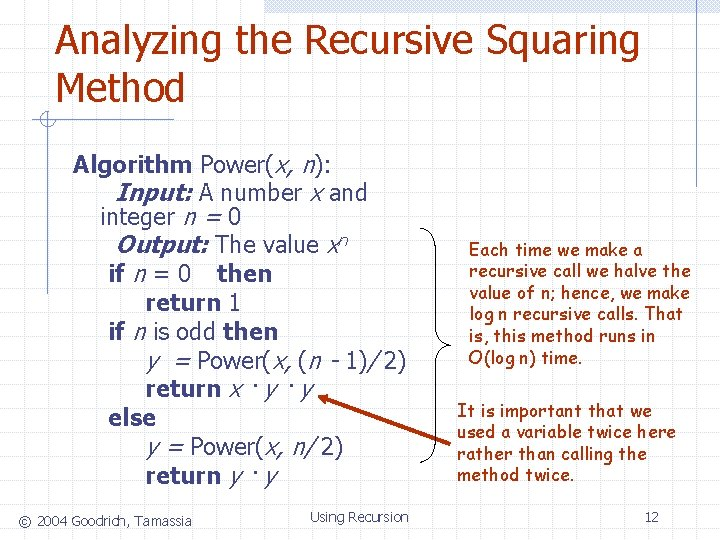Analyzing the Recursive Squaring Method Algorithm Power(x, n): Input: A number x and integer n = 0 Output: The value xn if n = 0 then return 1 if n is odd then y = Power(x, (n - 1)/ 2) return x · y else y = Power(x, n/ 2) return y · y © 2004 Goodrich, Tamassia Using Recursion Each time we make a recursive call we halve the value of n; hence, we make log n recursive calls. That is, this method runs in O(log n) time. It is important that we used a variable twice here rather than calling the method twice. 12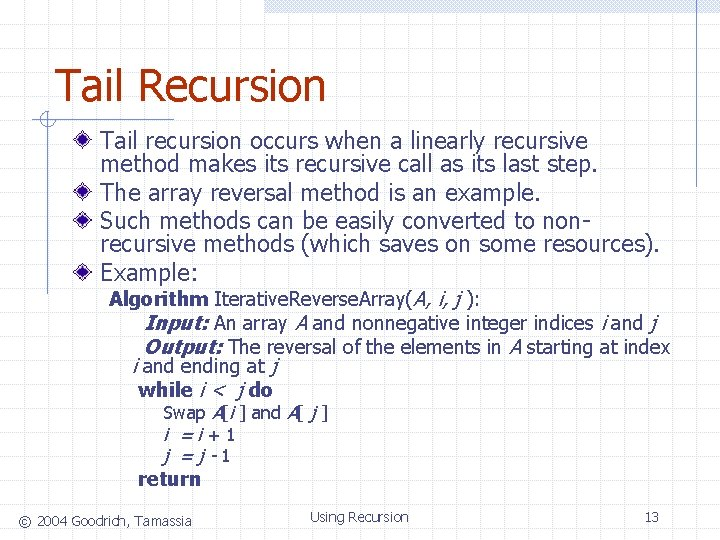Tail Recursion Tail recursion occurs when a linearly recursive method makes its recursive call as its last step. The array reversal method is an example. Such methods can be easily converted to nonrecursive methods (which saves on some resources). Example: Algorithm Iterative. Reverse. Array(A, i, j ): Input: An array A and nonnegative integer indices i and j Output: The reversal of the elements in A starting at index i and ending at j while i < j do Swap A[i ] and A[ j ] i =i+1 j =j-1 return © 2004 Goodrich, Tamassia Using Recursion 13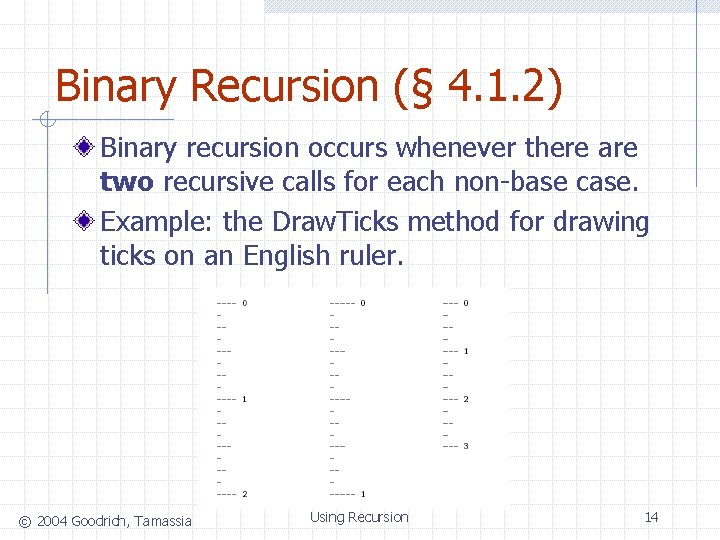Binary Recursion (§ 4. 1. 2) Binary recursion occurs whenever there are two recursive calls for each non-base case. Example: the Draw. Ticks method for drawing ticks on an English ruler. © 2004 Goodrich, Tamassia Using Recursion 14A Binary Recursive Method for Drawing Ticks // draw a tick with no label public static void draw. One. Tick(int tick. Length) { draw. One. Tick(tick. Length, - 1); } // draw one tick public static void draw. One. Tick(int tick. Length, int tick. Label) { for (int i = 0; i < tick. Length; i++) System. out. print("-"); if (tick. Label >= 0) System. out. print(" " + tick. Label); System. out. print("n"); } public static void draw. Ticks(int tick. Length) { // draw ticks of given length if (tick. Length > 0) { // stop when length drops to 0 draw. Ticks(tick. Length- 1); // recursively draw left ticks draw. One. Tick(tick. Length); // draw center tick draw. Ticks(tick. Length- 1); // recursively draw right ticks } } public static void draw. Ruler(int n. Inches, int major. Length) { // draw ruler draw. One. Tick(major. Length, 0); // draw tick 0 and its label for (int i = 1; i <= n. Inches; i++) { draw. Ticks(major. Length- 1); // draw ticks for this inch draw. One. Tick(major. Length, i); // draw tick i and its label } } © 2004 Goodrich, Tamassia Using Recursion Note the two recursive calls 15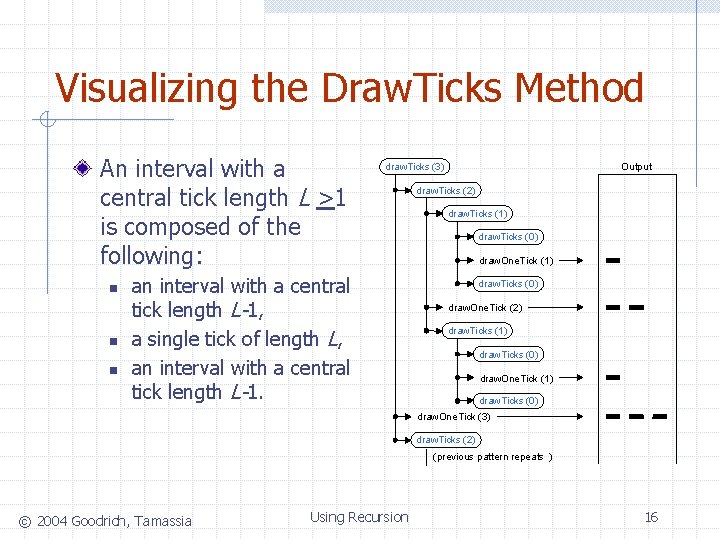Visualizing the Draw. Ticks Method An interval with a central tick length L >1 is composed of the following: n n n draw. Ticks (3) an interval with a central tick length L-1, a single tick of length L, an interval with a central tick length L-1. Output draw. Ticks (2) draw. Ticks (1) draw. Ticks (0) draw. One. Tick (3) draw. Ticks (2) (previous pattern repeats ) © 2004 Goodrich, Tamassia Using Recursion 16Another Binary Recusive Method Problem: add all the numbers in an integer array A: Algorithm Binary. Sum(A, i, n): Input: An array A and integers i and n Output: The sum of the n integers in A starting at index i if n = 1 then return A[i ] return Binary. Sum(A, i, n/ 2) + Binary. Sum(A, i + n/ 2, n/ 2) Example trace: 0, 8 0, 4 4, 4 0, 2 0, 1 2, 2 1, 1 © 2004 Goodrich, Tamassia 2, 1 4, 2 3, 1 4, 1 Using Recursion 6, 2 5, 1 6, 1 7, 1 17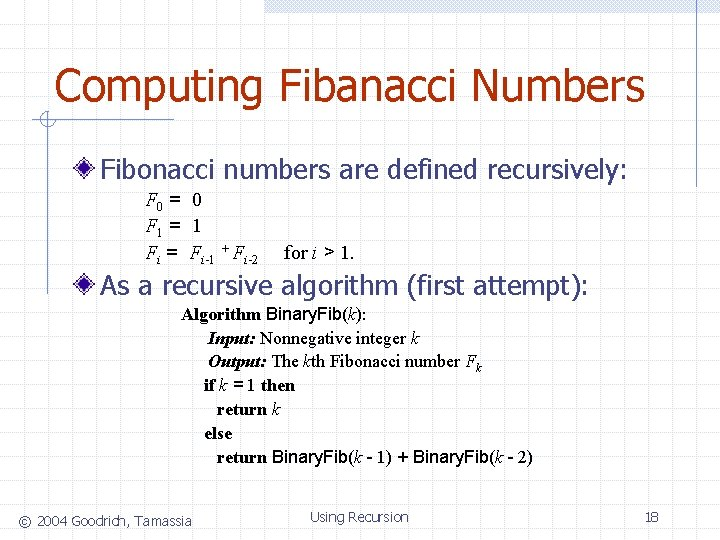Computing Fibanacci Numbers Fibonacci numbers are defined recursively: F 0 = 0 F 1 = 1 Fi = Fi-1 + Fi-2 for i > 1. As a recursive algorithm (first attempt): Algorithm Binary. Fib(k): Input: Nonnegative integer k Output: The kth Fibonacci number Fk if k = 1 then return k else return Binary. Fib(k - 1) + Binary. Fib(k - 2) © 2004 Goodrich, Tamassia Using Recursion 18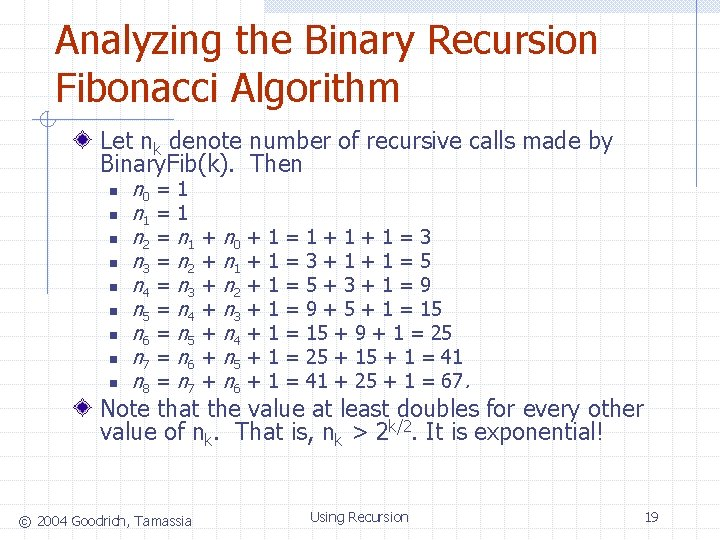Analyzing the Binary Recursion Fibonacci Algorithm Let nk denote number of recursive calls made by Binary. Fib(k). Then n n 0 = 1 n 1 = 1 n 2 = n 1 + n 0 + 1 = 1 + 1 = 3 n 3 = n 2 + n 1 + 1 = 3 + 1 = 5 n 4 = n 3 + n 2 + 1 = 5 + 3 + 1 = 9 n 5 = n 4 + n 3 + 1 = 9 + 5 + 1 = 15 n 6 = n 5 + n 4 + 1 = 15 + 9 + 1 = 25 n 7 = n 6 + n 5 + 1 = 25 + 1 = 41 n 8 = n 7 + n 6 + 1 = 41 + 25 + 1 = 67. Note that the value at least doubles for every other value of nk. That is, nk > 2 k/2. It is exponential! © 2004 Goodrich, Tamassia Using Recursion 19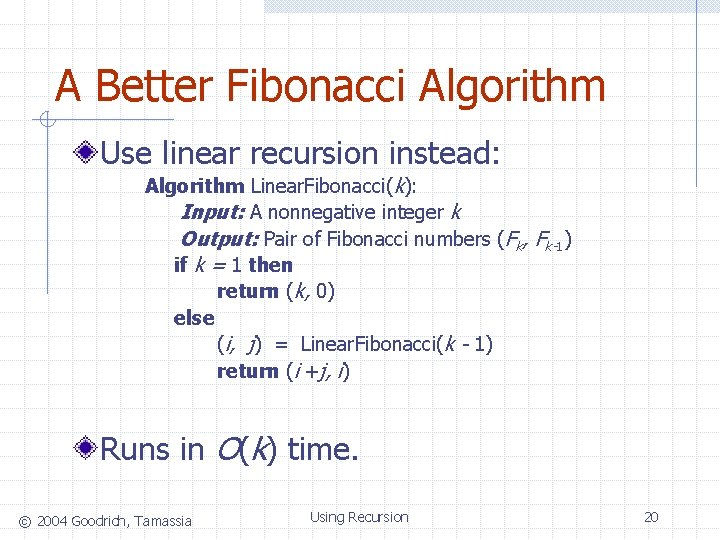A Better Fibonacci Algorithm Use linear recursion instead: Algorithm Linear. Fibonacci(k): Input: A nonnegative integer k Output: Pair of Fibonacci numbers (Fk, Fk-1) if k = 1 then return (k, 0) else (i, j) = Linear. Fibonacci(k - 1) return (i +j, i) Runs in O(k) time. © 2004 Goodrich, Tamassia Using Recursion 20Multiple Recursion (§ 4. 1. 3) Motivating example: summation puzzles w pot + pan = bib w dog + cat = pig w boy + girl = baby Multiple recursion: makes potentially many recursive calls (not just one or two). © 2004 Goodrich, Tamassia Using Recursion 21Algorithm for Multiple Recursion Algorithm Puzzle. Solve(k, S, U): Input: An integer k, sequence S, and set U (the universe of elements to test) Output: An enumeration of all k-length extensions to S using elements in U without repetitions for all e in U do Remove e from U {e is now being used} Add e to the end of S if k = 1 then Test whether S is a configuration that solves the puzzle if S solves the puzzle then return “Solution found: ” S else Puzzle. Solve(k - 1, S, U) Add e back to U {e is now unused} Remove e from the end of S © 2004 Goodrich, Tamassia Using Recursion 22Visualizing Puzzle. Solve Initial call Puzzle. Solve(3, (), {a, b, c}) Puzzle. Solve(2, b, {a, c}) Puzzle. Solve(2, a, {b, c}) Puzzle. Solve(1, ab, {c}) Puzzle. Solve(1, ba, {c}) abc bac Puzzle. Solve(2, c, {a, b}) Puzzle. Solve(1, ca, {b}) cab Puzzle. Solve(1, ac, {b}) Puzzle. Solve(1, bc, {a}) Puzzle. Solve(1, cb, {a}) acb bca cba © 2004 Goodrich, Tamassia Using Recursion 23